# Temperature field plots : finite element method

We introduced a finite element method to calculate the temperature fields.

Posted 2022-04-06
Categories: New feature

With the new version from March 2022, we provide two methods to calculate the approximated temperature field (ATF) for buried cables: The superposition principle and the Finite Element Method.

### Superposition principle

The original method to produce the approximated temperature field (ATF) for buried cables is based on the superposition principle. The temperature distribution is calculated assuming that the cables are directly buried in soil with a constant thermal resistivity $\rho_4$, that the heat loss $W_k$ is located in the center of cable or heat source $k$, and that the soil surface forms an isothermal at ambient temperature $\theta_a$.

$$\theta(x,y)=\theta_a+\frac{\rho_4}{2\pi}\sum\limits_{k=1}^N \left\{W_k\ln\left(\frac{\sqrt{(x-x_k)^2+(y+y_k)^2}}{\sqrt{(x-x_k)^2+(y-y_k)^2}}\right)\right\}$$

The temperature distribution does estimate the influence of the thermal resistivity of backfills $\rho_b$ if applicable. And in case of partial soil drying-out, the critical soil temperature $\theta_x$ is highlighted in red in the temperature field.

### Finite Element Method

With the new version from March 2022, a second method was introduced to calculate the approximated temperature distribution in the soil for buried, subsea, tunnels and for the first time even for troughs. This new method is using the finite elements to calculate the heat distribution through conduction using the calculated conductor temperatures as boundary condition.

ATF with colormap coolwarmMesh field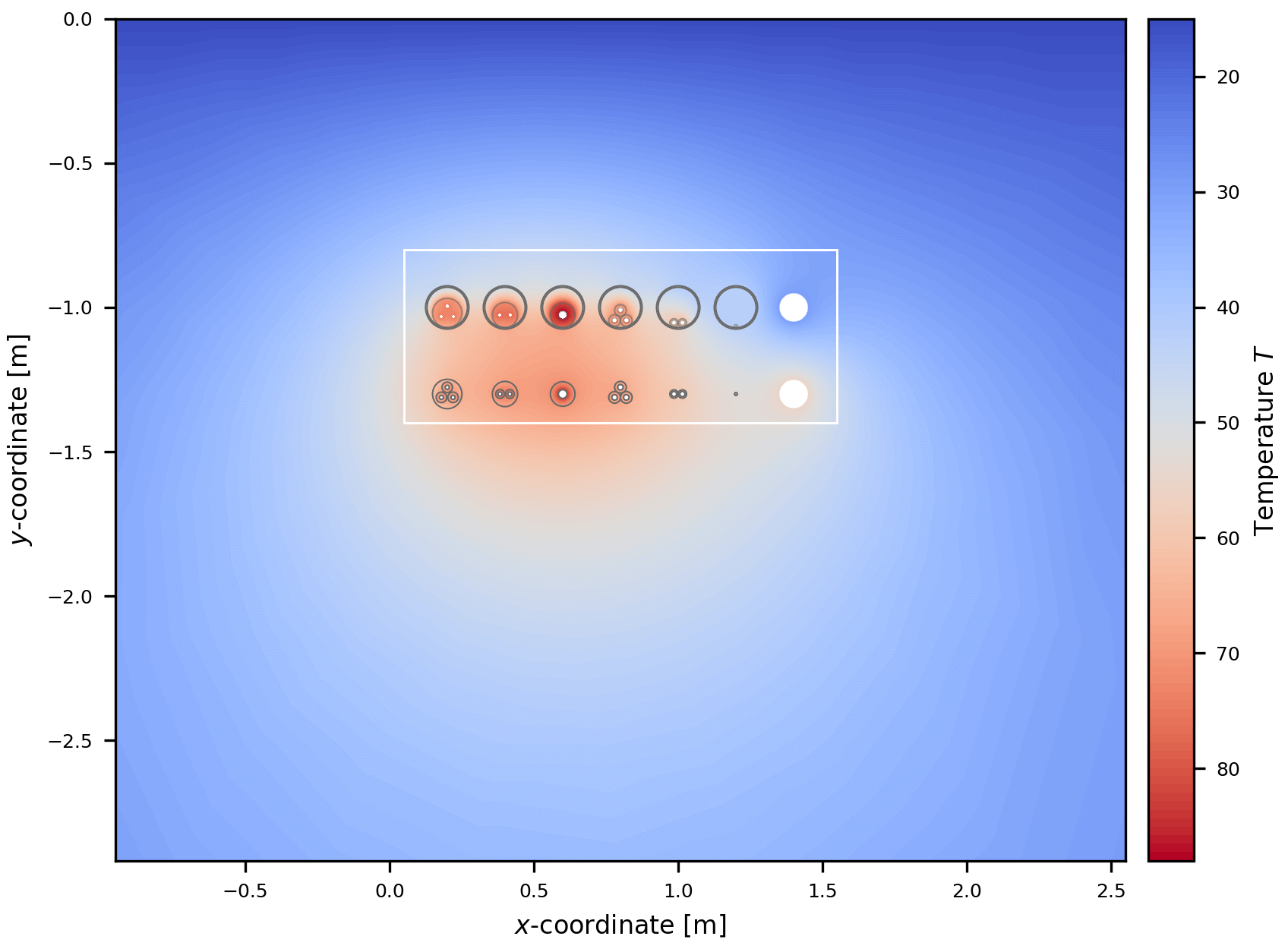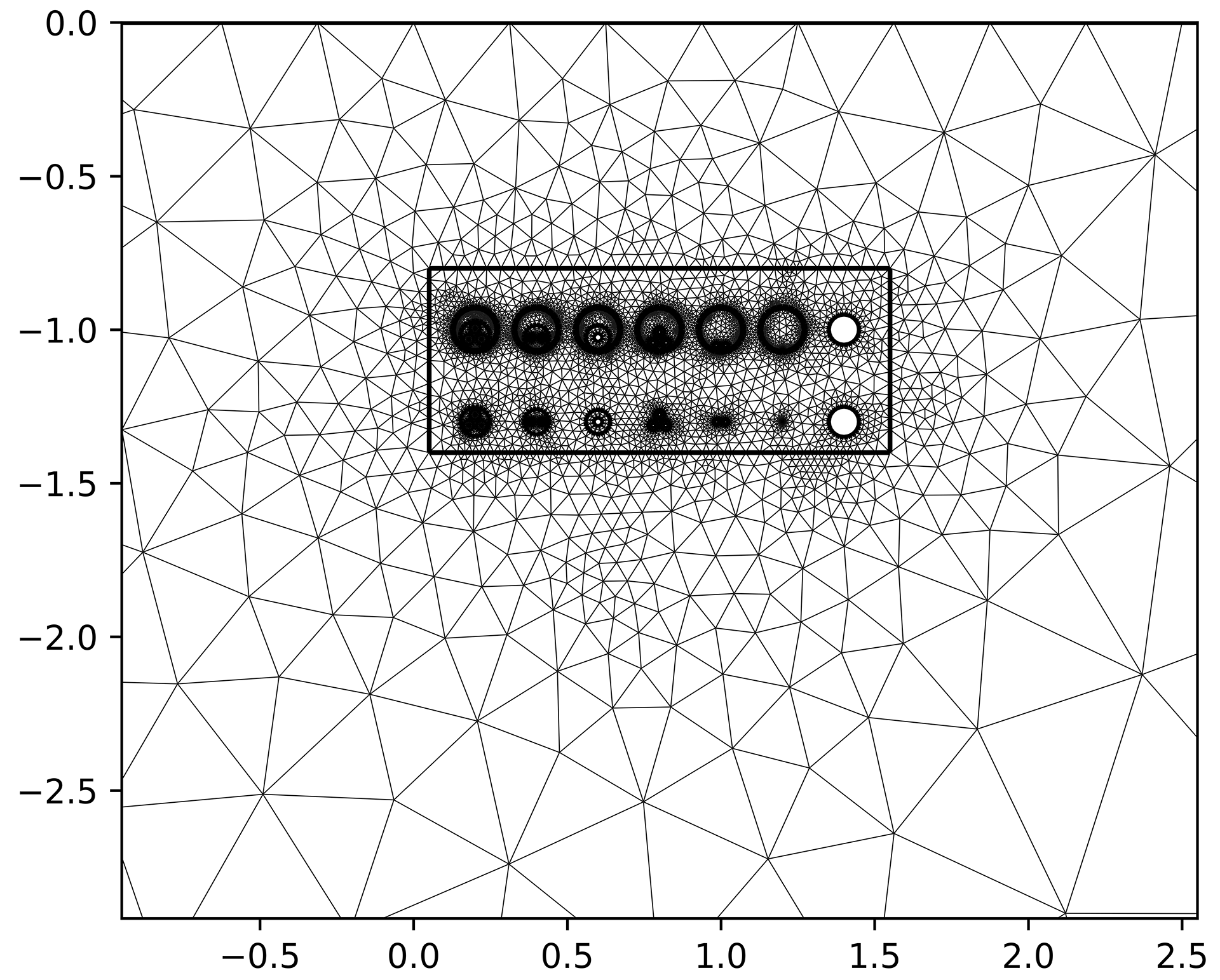The extensive python FEM module PyGimli is used for all our applications using the finite element method, including the calculation of the temperature distribution. Thanks to the contributors of PyGimli.

The radio selector to choose finite element method to create the ATF had been there since mid December 2021, but except for very simple installations, it was unfortunately not working properly yet. The now deployed version is working stable in all tested cases.

#### Definitions

Following definitions of the finite element model apply:

1. The geometry is defined within a world with a range of at least 15 m to the sides and below.
2. The soil surface and the objects surfaces are Dirichlet boundary conditions (BC) with the ambient temperature $\theta_a$ and the surface temperatures of the object $\theta_e$ as a result from the ampacity calculation.
3. The bottom and the left and right sides are natural BC, meaning they are of homogeneous Neumann type $∂u/∂n=0$, also called adiabatic or perfectly insulated BC.
4. As we are calculating steady-state conditions, diffusivity in the pygimli slver is replaced with conductivity $q=-k · div(T)$.
5. The soil is modelled with the given thermal conductivtiy $k_{4}=1/\rho_{4}$ and backfill areas are modelled with their given, corresponding thermal conductivity $k_{b}=1/\rho_{b}$.
6. The duct walls, cable jackets and cable insulation layers are modelled with a typical thermal conductivity $k_{ins}=0.3$; conductors are modelled with a thermal conductivty of $k_{ins}=300$ (between copper and aluminium); screen, sheath, armour and steel pipes are not modelled; for air inside ducts the typical thermal conductivity of air at 50 °C; is used (or of bentonite).
7. In case of non-homogenous soil surface and for troughs, the geometry is being extended into the airspace above the surface and a typical thermal conductivity of air at 25 °C is used. In case of subsea, the same is done with water instead of air.

The following images show how the geometry is defined within a world with a range of 20 m to the sides and below.

ATF of world geometryMesh field of world geometry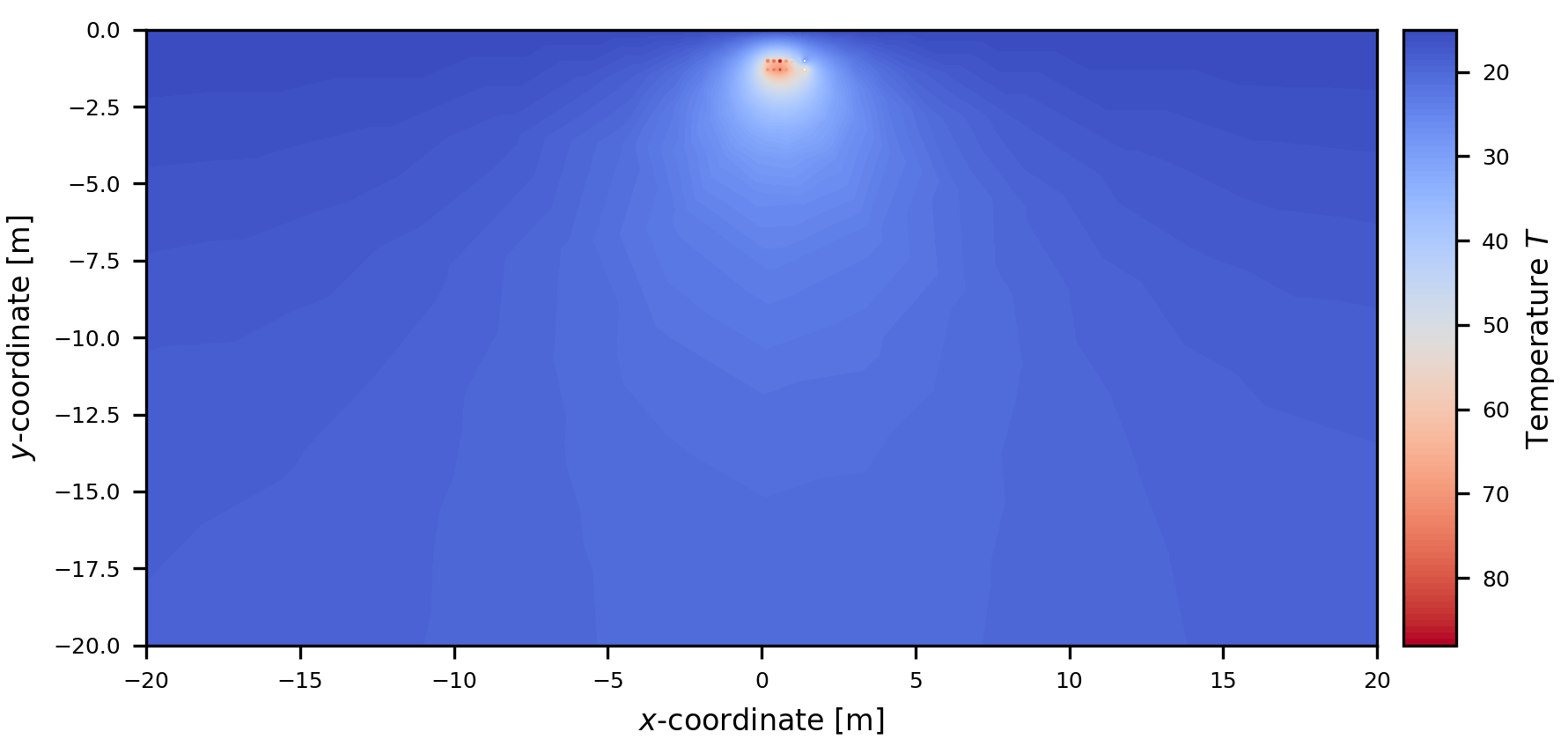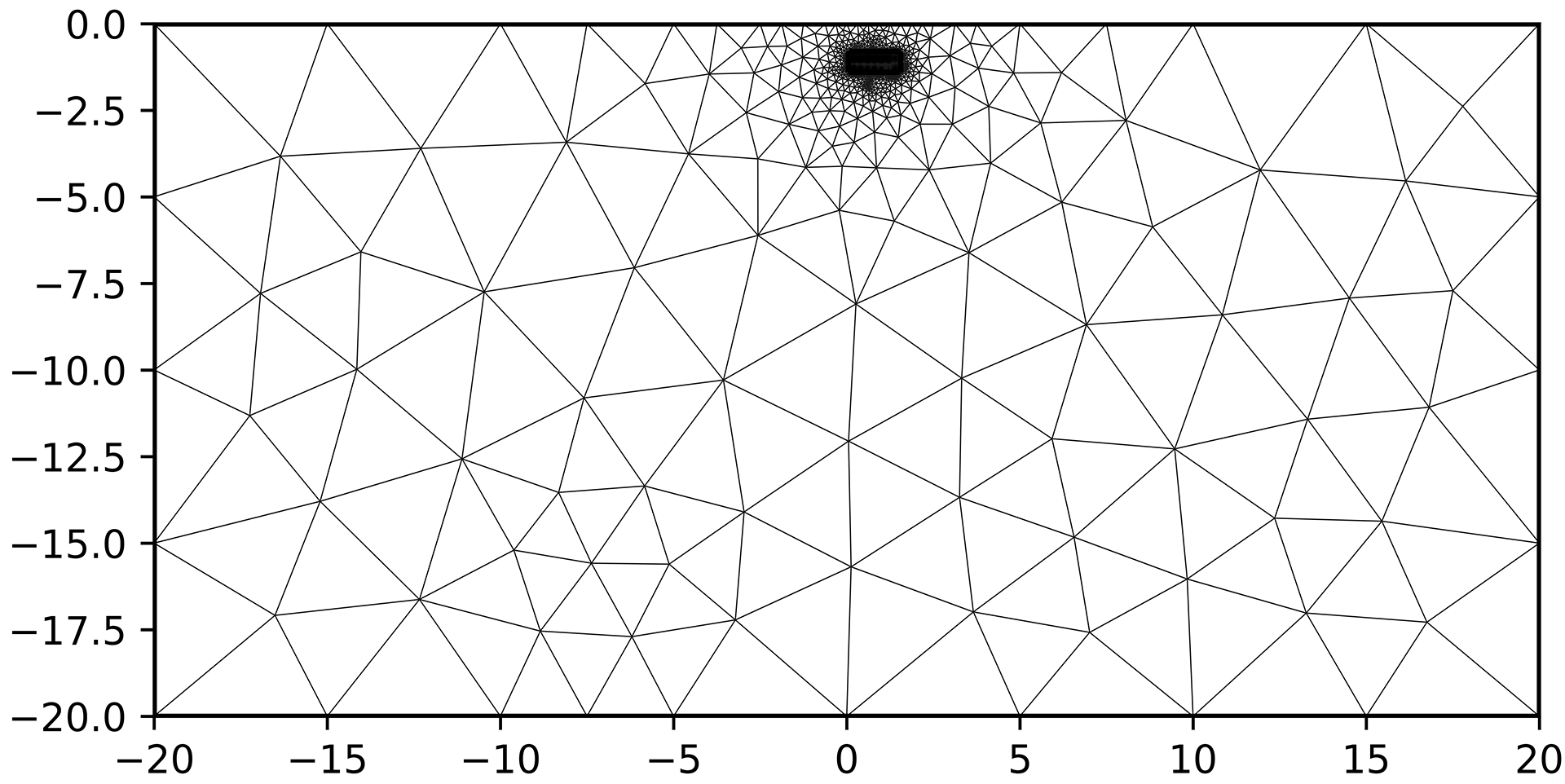#### Features

This new method comes with following features which will provide a fantastic user experience.

• Include the plot directly into the report or recalculate it on the result page and download png file for further use.
• Show the temperature distribution within cables.
• Show the temperature distribution within air- and bentonite-filled ducts.
• Show the temperature distribution within troughs and tunnels based.
• Define minimal and maximal limits of the colormesh.
• Set the $\Delta\theta$ stepsize for the colormesh with a minimal stepsize of 0.1 °C
• Choose from 20 different Matplotlib colormaps plus optionally reverse them.

Limitations are:

• Heat flow within conductors is not considered, the surface is a Dirichlet boundary condition (temperature).
• Heat flow within heat sources is not considered as the interior is unknown.
• No visualization of isolines is possible, please use a larger stepsize for $\Delta\theta$.
• Temperature distribution within troughs and tunnels does not consider convection.
• To improve the stiffness stability of the mesh, backfills will typically be modelled with rounded corners.

Following plots show fine details of the plot and the mesh field. This shall bear witness to the outstanding properties of this new method.

Details of the ATF with colormap plasmaDetailes of the mesh field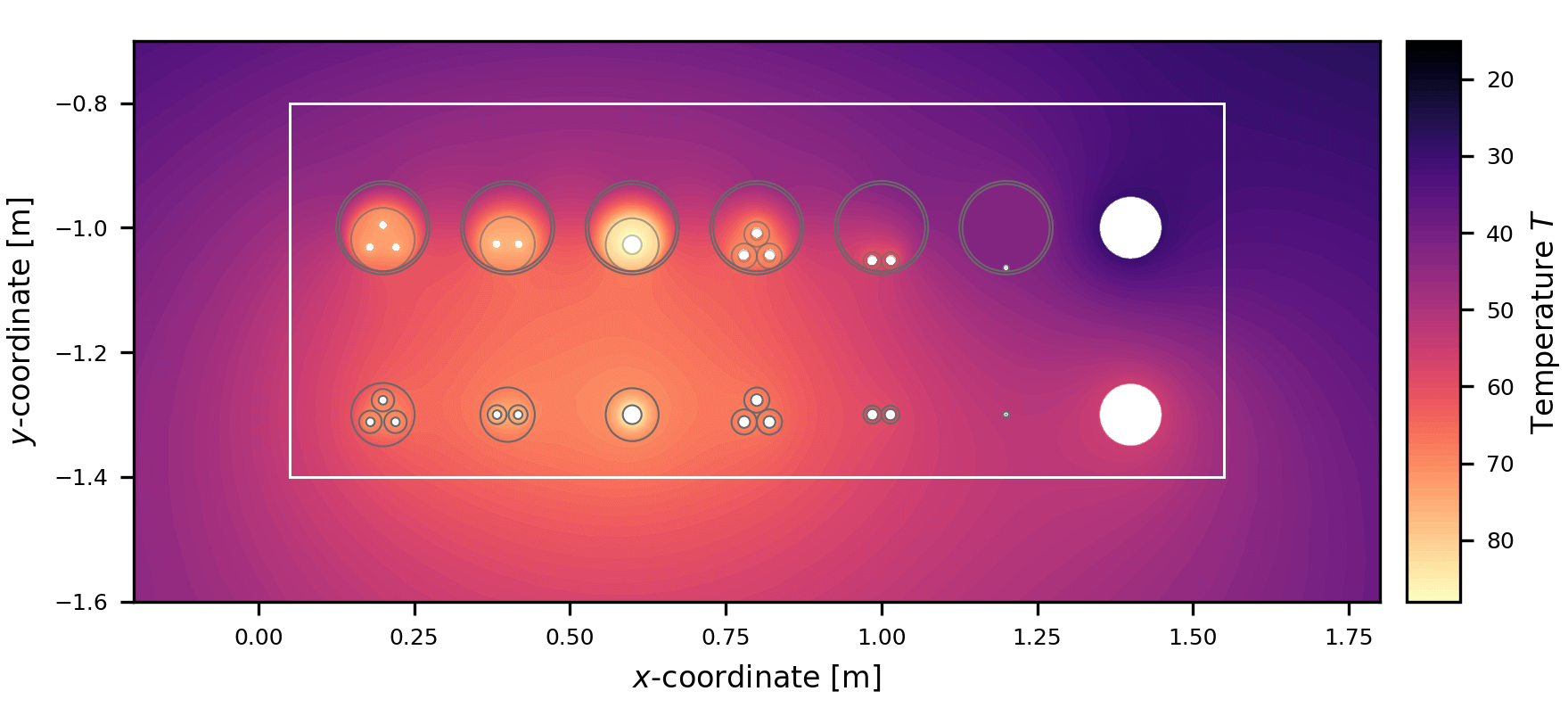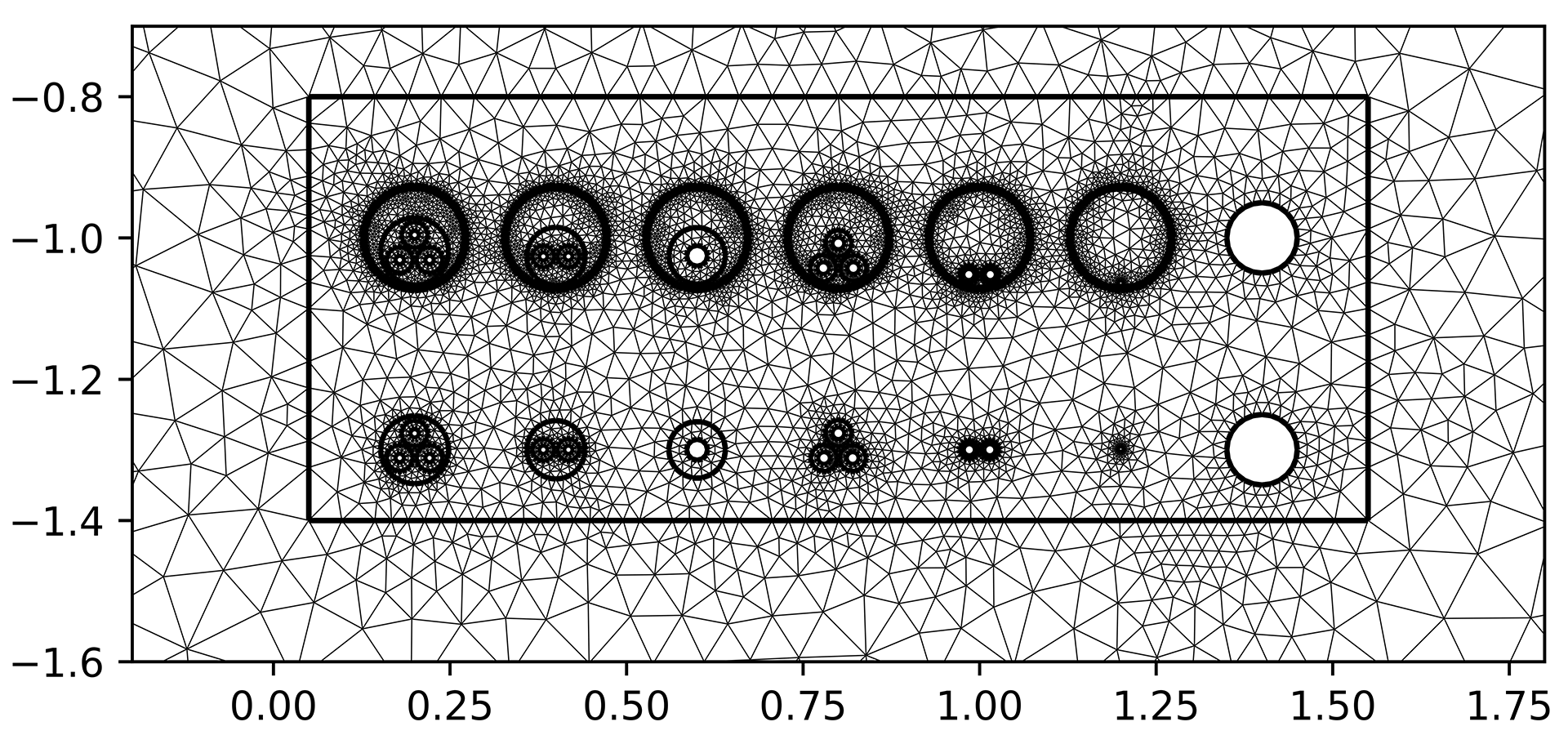Based on the implemented finite element method for ATF, we will introduce the possibility to do the thermal rating calculation using FEM for buried cables. This feature will come in the near future and will allow the users to accurately consider the effect of non-homogenous environment.

6
25
12
24
10
9
10
2
9
11
2